Lfant Lithe and Fast Algorithmic Number Theory Algorithmics, Programming, Software and Architecture Algorithms, Certification, and Cryptography

Lfantis an INRIA project-team joint with University of Bordeaux and CNRS (IMB, UMR 5251). The team was created on March 1st, 2009, and has become a project-team on January 1st, 2010.

Andreas Enge INRIA Chercheur Bordeaux Team leader, INRIA Research Director oui Karim Belabas UnivFr Enseignant Bordeaux Professor, University Bordeaux 1 oui Jean-Paul Cerri UnivFr Enseignant Bordeaux Associate professor, University Bordeaux 1 Henri Cohen UnivFr Enseignant Bordeaux Professor emeritus, University Bordeaux 1 oui Jean-Marc Couveignes INRIA Enseignant Bordeaux Professor, on leave from University of Toulouse II oui Bill Allombert CNRS Technique Bordeaux CNRS, since 05/2010 Patricia Maleyran INRIA Assistant Bordeaux Jean-François Biasse CNRS PhD Bordeaux DGA-CNRS, until 09/2010 Pierre Lezowski UnivFr PhD Bordeaux ENS, since 09/2009 Jérôme Milan CNRS PhD Bordeaux ANR, since 09/2009 Pascal Molin UnivFr PhD Bordeaux ENS, until 09/2010; ATER University Bordeaux 1, 10/2010–11/2010 Vincent Verneuil EtablissementPrive PhD Bordeaux CIFRE Inside Contactless, since 2009 Anna Morra UnivFr PostDoc Bordeaux ATER University Bordeaux 1, until 09/2010 Damien Robert INRIA PostDoc Bordeaux INRIA, since 10/2010 Pieter Rozenhart INRIA PostDoc Bordeaux INRIA, 01/2010–12/2010 Overall Objectives Presentation

Algorithmic number theory dates back to the dawn of mathematics itself, cf.Eratosthenes's sieve to enumerate consecutive prime numbers. With the arrival of computers, previously unsolvable problems have come into reach, which has boosted the development of more or less practical algorithms for essentially all number theoretic problems. The field is now mature enough for a more computer science driven approach, taking into account the theoretical complexities and practical running times of the algorithms.

Concerning the lower level multiprecision arithmetic, folklore has asserted for a long time that asymptotically fast algorithms such as Schönhage–Strassen multiplication are impractical; nowadays, however, they are used routinely. On a higher level, symbolic computation provides numerous asymptotically fast algorithms (such as for the simultaneous evaluation of a polynomial in many arguments or linear algebra on sparse matrices), which have only partially been exploited in computational number theory. Moreover, precise complexity analyses do not always exist, nor do sound studies to choose between different algorithms (an exponential algorithm may be preferable to a polynomial one for a large range of inputs); folklore cannot be trusted in a fast moving area such as computer science.

Another problem is the reliability of the computations; many number theoretic algorithms err with a small probability, depend on unknown constants or rely on a Riemann hypothesis. The correctness of their output can either be ensured by a special design of the algorithm itself (slowing it down) or by an a posterioriverification. Ideally, the algorithm outputs a certificate, providing an independent fastcorrectness proof. An example is integer factorisation, where factors are hard to obtain but trivial to check; primality proofs have initiated sophisticated generalisations.

One of the long term goals of the Lfantproject team is to make an inventory of the major number theoretic algorithms, with an emphasis on algebraic number theory and arithmetic geometry, and to carry out complexity analyses. So far, most of these algorithms have been designed and tested over number fields of small degree and scale badly. A complexity analysis should naturally lead to improvements by identifying bottlenecks, systematically redesigning and incorporating modern asymptotically fast methods.

Reliability of the developed algorithms is a second long term goal of our project team. Short of proving the Riemann hypothesis, this could be achieved through the design of specialised, slower algorithms not relying on any unproven assumptions. We would prefer, however, to augment the fastest unproven algorithms with the creation of independently verifiable certificates. Ideally, it should not take longer to check the certificate than to generate it.

All theoretical results are complemented by concrete reference implementations in Pari/Gp, which allow to determine and tune the thresholds where the asymptotic complexity kicks in and help to evaluate practical performances on problem instances provided by the research community. Another important source for algorithmic problems treated by the Lfantproject team is modern cryptology. Indeed, the security of all practically relevant public key cryptosystems relies on the difficulty of some number theoretic problem; on the other hand, implementing the systems and finding secure parameters require efficient algorithmic solutions to number theoretic problems.

Highlights

P. Molin has defended his PhD thesis on “Intégration numérique et calculs de fonctions L. J.-F. Biasse has defended his PhD thesis on “Subexponential algorithms for number fields” . In May, A. Enge has given the science talk at the ceremony awarding the Abel Prize to John Tate in Oslo. The talk, entitled “The queen of mathematics in communication security”, presented links between Tate's work and cryptologic applications.

Scientific Foundations Number fields, class groups and other invariants Bill Allombert Karim Belabas Jean-François Biasse Jean-Paul Cerri Henri Cohen Jean-Marc Couveignes Andreas Enge Pierre Lezowski Pascal Molin Anna Morra

Modern number theory has been introduced in the second half of the 19th century by Dedekind, Kummer, Kronecker, Weber and others, motivated by Fermat's conjecture: There is no non-trivial solution in integers to the equation xn+ yn= znfor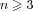. For recent textbooks, see . Kummer's idea for solving Fermat's problem was to rewrite the equation as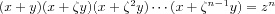for a primitive n-th root of unity, which seems to imply that each factor on the left hand side is an n-th power, from which a contradiction can be derived.

The solution requires to augment the integers by algebraic numbers, that are roots of polynomials in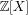. For instance,is a root of Xn-1,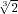is a root of X3-2and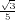is a root of 25 X 2-3. A number fieldconsists of the rationals to which have been added finitely many algebraic numbers together with their sums, differences, products and quotients. It turns out that actually one generator suffices, and any number field Kis isomorphic to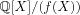, where f( X)is the minimal polynomial of the generator. Of special interest are algebraic integers, “numbers without denominators”, that are roots of a monic polynomial. For instance,andare integers, whileis not. The ring of integersof Kis denoted by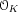; it plays the same role in Kas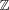in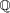.

Unfortunately, elements inmay factor in different ways, which invalidates Kummer's argumentation. Unique factorisation may be recovered by switching to ideals, subsets ofthat are closed under addition and under multiplication by elements of. In, for instance, any ideal is principal, that is, generated by one element, so that ideals and numbers are essentially the same. In particular, the unique factorisation of ideals then implies the unique factorisation of numbers. In general, this is not the case, and the class group Cl Kof ideals ofmodulo principal ideals and its class number hK= |Cl K|measure how faris from behaving like.

Using ideals introduces the additional difficulty of having to deal with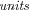, the invertible elements of: Even when hK= 1, a factorisation of ideals does not immediately yield a factorisation of numbers, since ideal generators are only defined up to units. For instance, the ideal factorisation (6) = (2)·(3)corresponds to the two factorisations 6 = 2·3and 6 = (-2)·(-3). While in, the only units are 1 and -1, the unit structure in general is that of a finitely generated-module, whose generators are the fundamental units. The regulator RKmeasures the “size” of the fundamental units as the volume of an associated lattice.

One of the main concerns of algorithmic algebraic number theory is to explicitly compute these invariants ( Cl Kand hK, fundamental units and RK), as well as to provide the data allowing to efficiently compute with numbers and ideals of; see for a recent account.

The analytic class number formulalinks the invariants hKand RK(unfortunately, only their product) to the-function of K,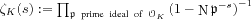, which is meaningful when( s)>1, but which may be extended to arbitrary complex s1. Introducing characters on the class group yields a generalisation of- to L-functions. The generalised Riemann hypothesis (GRH), which remains unproved even over the rationals, states that any such L-function does not vanish in the right half-plane( s)>1/2. The validity of the GRH has a dramatic impact on the performance of number theoretic algorithms. For instance, under GRH, the class group admits a system of generators of polynomial size; without GRH, only exponential bounds are known. Consequently, an algorithm to compute Cl Kvia generators and relations (currently the only viable practical approach) either has to assume that GRH is true or immediately becomes exponential.

When hK= 1the number field Kmay be norm-Euclidean, endowingwith a Euclidean division algorithm. This question leads to the notions of the Euclidean minimum and spectrum of K, and another task in algorithmic number theory is to compute explicitly this minimum and the upper part of this spectrum, yielding for instance generalised Euclidean gcd algorithms.

Function fields, algebraic curves and cryptology Karim Belabas Jean-François Biasse Jean-Marc Couveignes Andreas Enge Jérôme Milan Pascal Molin Damien Robert Pieter Rozenhart Vincent Verneuil

Algebraic curves over finite fields are used to build the currently most competitive public key cryptosystems. Such a curve is given by a bivariate equation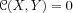with coefficients in a finite field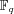. The main classes of curves that are interesting from a cryptographic perspective are elliptic curvesof equation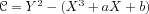and hyperelliptic curvesof equationwith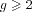.

The cryptosystem is implemented in an associated finite abelian group, the Jacobian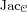. Using the language of function fields exhibits a close analogy to the number fields discussed in the previous section. Let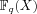(the analogue of) be the rational function fieldwith subring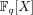(which is principal just as). The function fieldofis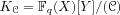; it contains the coordinate ring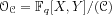. Definitions and properties carry over from the number field case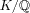to the function field extension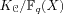. The Jacobianis the divisor class group of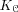, which is an extension of (and for the curves used in cryptography usually equals) the ideal class group of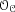.

The size of the Jacobian group, the main security parameter of the cryptosystem, is given by an L-function. The GRH for function fields, which has been proved by Weil, yields the Hasse–Weil bound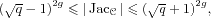or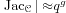, where the genus gis an invariant of the curve that correlates with the degree of its equation. For instance, the genus of an elliptic curve is 1, that of a hyperelliptic one is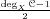. An important algorithmic question is to compute the exact cardinality of the Jacobian.

The security of the cryptosystem requires more precisely that the discrete logarithm problem(DLP) be difficult in the underlying group; that is, given elements D1and D2= xD1of, it must be difficult to determine x. Computing xcorresponds in fact to computingexplicitly with an isomorphism to an abstract product of finite cyclic groups; in this sense, the DLP amounts to computing the class group in the function field setting.

For any integer n, the Weil pairing enonis a function that takes as input two elements of order nofand maps them into the multiplicative group of a finite field extension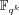with k= k( n)depending on n. It is bilinear in both its arguments, which allows to transport the DLP from a curve into a finite field, where it is potentially easier to solve. The Tate-Lichtenbaum pairing, that is more difficult to define, but more efficient to implement, has similar properties. From a constructive point of view, the last few years have seen a wealth of cryptosystems with attractive novel properties relying on pairings.

For a random curve, the parameter kusually becomes so big that the result of a pairing cannot even be output any more. One of the major algorithmic problems related to pairings is thus the construction of curves with a given, smallish k.

Complex multiplication Karim Belabas Henri Cohen Jean-Marc Couveignes Andreas Enge Damien Robert

Complex multiplication provides a link between number fields and algebraic curves; for a concise introduction in the elliptic curve case, see Sect. 1.1, for more background material, . In fact, for most curvesover a finite field, the endomorphism ring of, which determines its L-function and thus its cardinality, is an order in a special kind of number field K, called CM field. The CM field of an elliptic curve is an imaginary-quadratic fieldwith D<0, that of a hyperelliptic curve of genus  gis an imaginary-quadratic extension of a totally real number field of degree  g. Deuring's lifting theorem ensures thatis the reduction modulo some prime of a curve with the same endomorphism ring, but defined over the Hilbert class field HKof K.

Algebraically, HKis defined as the maximal unramified abelian extension of K; the Galois group of HK/ Kis then precisely the class group Cl K. A number field extension H/ Kis called Galoisif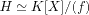and Hcontains all complex roots of  f. For instance,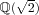is Galois since it contains not only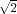, but also the second root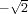of X2-2, whereas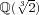is not Galois, since it does not contain the root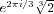of X3-2. The Galois group Gal H/ Kis the group of automorphisms of Hthat fix K; it permutes the roots of  f. Finally, an abelianextension is a Galois extension with abelian Galois group.

Analytically, in the elliptic case HKmay be obtained by adjoining to Kthe singular value j()for a complex valued, so-called modularfunction jin some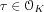; the correspondence between Gal H/ Kand Cl Kallows to obtain the different roots of the minimal polynomial fof j()and finally fitself. A similar, more involved construction can be used for hyperelliptic curves. This direct application of complex multiplication yields algebraic curves whose L-functions are known beforehand; in particular, it is the only possible way of obtaining ordinary curves for pairing-based cryptosystems.

The same theory can be used to develop algorithms that, given an arbitrary curve over a finite field, compute its L-function.

A generalisation is provided by ray class fields; these are still abelian, but allow for some well-controlled ramification. The tools for explicitly constructing such class fields are similar to those used for Hilbert class fields.

Application Domains Number theory

Being able to compute quickly and reliably algebraic invariants is an invaluable aid to mathematicians: It fosters new conjectures, and often shoots down the too optimistic ones. Moreover, a large body of theoretical results in algebraic number theory has an asymptotic nature and only applies for large enough inputs; mechanised computations (preferably producing independently verifiable certificates) are often necessary to finish proofs.

For instance, many Diophantine problems reduce to a set of Thue equations of the form P( x, y) = afor an irreducible, homogeneous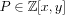,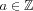, in unknown integers x, y. In principle, there is an algorithm to solve the latter, provided the class group and units of a rupture field of Pare known. Since there is no other way to prove that the full set of solutions is obtained, these algebraic invariants must be computed and certified, preferably without using the GRH.

Deeper invariants such as the Euclidean spectrum are related to more theoretical concerns, e.g., determining new examples of principal, but not norm-Euclidean number fields, but could also yield practical new algorithms: Even if a number field has class number larger than 1 (in particular, it is not norm-Euclidean), knowing the upper part of the spectrum should give a partialgcd algorithm, succeeding for almost all pairs of elements of. As a matter of fact, every number field which is not a complex multiplication field and whose unit group has rank strictly greater than 1 is almost norm-Euclidean  , .

Algorithms developed by the team are implemented in the free Pari/Gpsystem for number theory maintained by K. Belabas, which is a reference and the tool of choice for the worldwide number theory community.

Cryptology

Public key cryptology has become a major application domain for algorithmic number theory. This is already true for the ubiquitous RSA system, but even more so for cryptosystems relying on the discrete logarithm problem in algebraic curves over finite fields . For the same level of security, the latter require smaller key lengths than RSA, which results in a gain of bandwidth and (depending on the precise application) processing time. Especially in environments that are constrained with respect to space and computing power such as smart cards and embedded devices, algebraic curve cryptography has become the technology of choice. Most of the research topics of the Lfantteam concern directly problems relevant for curve-based cryptology: The difficulty of the discrete logarithm problem in algebraic curves determines the security of the corresponding cryptosystems. Complex multiplication, point counting and isogenies provide, on one hand, the tools needed to create secure instances of curves. On the other hand, isogenies have been found to have direct cryptographic applications to hash functions and encryption . Pairings in algebraic curves have proved to be a rich source for novel cryptographic primitives. Class groups of number fields also enter the game as candidates for algebraic groups in which cryptosystems can be implemented. However, breaking these systems by computing discrete logarithms has proved to be easier than in algebraic curves; we intend to pursue this cryptanalytic strand of research.

Apart from solving specific problems related to cryptology, number theoretic expertise is vital to provide cryptologic advice to industrial partners in joint projects. It is to be expected that continuing pervasiveness and ubiquity of very low power computing devices will render the need for algebraic curve cryptography more pressing in coming years.

Software Pari/Gp Karim Belabas correspondant Bill Allombert Henri Cohen Andreas Enge

http:// pari. math. u-bordeaux. fr/

Current stable version of Pari/Gp: 2.3.5, 2010

Current testing version of Pari/Gp: 2.4.3.alpha, 2010

Current version of gp2c: 0.0.5pl10, 2010

Pari/Gpis a widely used computer algebra system designed for fast computations in number theory (factorisation, algebraic number theory, elliptic curves, ...), but it also contains a large number of other useful functions to compute with mathematical entities such as matrices, polynomials, power series, algebraic numbers, etc., and many transcendental functions.

• Pariis a C library, allowing fast computations.

• gp2c, the GP-to-C compiler, combines the best of both worlds by compiling Gpscripts to the C language and transparently loading the resulting functions into Gp; scripts compiled by gp2cwill typically run three to four times faster.

• Mpc Andreas Enge correspondant Philippe Théveny Paul Zimmermann Inriaproject-team Caramel

http:// mpc. multiprecision. org/

Current version: 0.8.2 Dianthus deltoides, 2010

Mpcis a C library for the arithmetic of complex numbers with arbitrarily high precision and correct rounding of the result. It is built upon and follows the same principles as Mpfr. The Mpclibrary has been registered in France by the Agence pour la Protection des Programmes on 2003-02-05 under the number IDDN FR 001 060029 000 R P 2003 000 10000.

It is a prerequisite for the release 4.5 of the Gnucompiler collection Gcc, where it is used in the C and Fortran frontends for constant folding, the evaluation of constant mathematical expressions during the compilation of a program.

Mpfrcx Andreas Enge

http:// mpfrcx. multiprecision. org/

Current version: 0.3.1 Banane, 2010

Mpfrcxis a library for the arithmetic of univariate polynomials over arbitrary precision real ( Mpfr) or complex ( Mpc) numbers, without control on the rounding. For the time being, only the few functions needed to implement the floating point approach to complex multiplication are implemented. On the other hand, these comprise asymptotically fast multiplication routines such as Toom-Cook and the FFT.

Cm Andreas Enge

http:// cm. multiprecision. org/

Initial public release: version 0.1 Apfelkraut, 2009

The Cmsoftware implements the construction of ring class fields of imaginary quadratic number fields and of elliptic curves with complex multiplication via floating point approximations. It consists of libraries that can be called from within a C program and of executable command line applications. For the implemented algorithms, see .

Cubic Karim Belabas

http:// www. math. u-bordeaux. fr/ ~belabas/ research/ software/ cubic-1. 0. tgz

Current stable version: 1.0, 2009

Cubicis a standalone program that prints out generating equations for cubic fields of either signature and bounded discriminant. It depends on the Parilibrary. The algorithm has quasi-linear time complexity in the size of the output.

New Results Discrete logarithms Andreas Enge

In , we presented for the first time an algorithm for the discrete logarithm problem in certain algebraic curves that runs in subexponential time less than L(1/2), namely, L(1/3 +)for any>0. In , we lower this complexity to L(1/3), showing that the corresponding algebraic curves (essentially Cabcurves of genus ggrowing at least quadratically with the logarithmic size of the finite field of definition, log q) result in cryptosystems that are as easily attacked as RSA or tradtional cryptosystems based on discrete logarithms in finite fields. We provide a complete classification of all the curves to which the attack applies. The article has been accepted by Journal of Cryptology.

Class groups and other invariants of number fields Bill Allombert Karim Belabas Jean-François Biasse Jean-Paul Cerri Pierre Lezowski

J.-F. Biasse has made practical improvements to the sieving-based algorithm of Jacobson for computing the group structure of the ideal class group of an imaginary-quadratic number field . These improvements, based on the use of large prime variations combined with structured Gaussian elimination, have led to the computation of the class group structure of a number field with a 110-digit discriminant (whereas older techniques were limited to 90-digit discriminants).

He has also determined a class of number fields for which the ideal class group, the regulator, and a system of fundamental units of the maximal order can be computed in subexponential time L(1/3, O(1))(whereas the best previously known algorithms have complexity L(1/2, O(1))). This class of number fields is analogous to the class of curves described in , cf. . The article has been submitted to Mathematics of Computation.

In collaboration with M. Jacobson, J.-F. Biasse has described improvements to the sieving methods for ideal class group, regulator and fundamental unit computation . These improvements lead to a significant speed-up over the previous state of the art, and the computation of the regulator of a number field of a 110 digit discriminant, whereas the previous record was 100 digits.

Together with M. Jacobson and A. Silvester, J.-F. Biasse has improved the algorithms for solving the discrete logarithm problem and the principal ideal problem, which are involved in the design of cryptosystems based on number fields . They have assessed the impact of these improvements on the security of theses cryptosystems and provided estimates on the size of the keys required to ensure a level of security equivalent to the recommendations of the NIST.

Using new theoretical ideas and his novel algorithmic approach, J.-P. Cerri has discovered examples of generalised Euclidean number fields and of 2-stage norm-Euclidean number fields in degree greater than 2  . These notions, extending the link between usual Euclideanity and principality of the ring of integers of a number field had already received much attention before; however, examples were only known for quadratic fields.

The algorithms developed by J.-P. Cerri for totally real fields are currently being generalised by P. Lezowski to arbitrary number fields.

In collaboration with L. Grenié, B. Allombert and K. Belabas have considerably improved the practical efficiency of PARI/GP algorithms computing class and unit groups, in particular for number fields of large degree. They are currently working on a flexible parallelisation framework of the PARI/GP system; a first application target is to improve the relation finding part of the class group algorithms (affording in principle a linear speedup).

Number and function field enumeration Karim Belabas Henri Cohen Anna Morra Pieter Rozenhart

In joint work with É. Fouvry (Orsay), K. Belabas has proved a new case of Malle's conjecture, a strong effective form of the inverse Galois problem  . They have given an asymptotic enumeration of Galois sextic fields with group S3, ordered by discriminant, using classical Davenport-Heilbronn theory in a novel way. The same result was independently obtained by Bhargava and Wood using a different method.

In joint work with R. Scheidler and M. Jacobson, P. Rozenhart has generalized Belabas's algorithm for tabulating cubic number fields to cubic function fields. This generalization required function field analogues of the Davenport-Heilbronn Theorem and of the reduction theory of binary cubic and quadratic forms. As an additional application, they have modified the tabulation algorithm to compute 3-ranks of quadratic function fields by way of a generalisation of a theorem due to Hasse. The algorithm, whose complexity is quasi-linear in the number of reduced binary cubic forms up to some upper bound X, works very well in practice. The article has been submitted to Mathematics of Computation. A follow-up article describes how to use these results to compute 3-ranks of quadratic function fields, in particular yielding examples of unusually high 3-rank.

H. Cohen and A. Morra have obtained an explicit expression for the Dirichlet generating function associated to cubic extensions of an arbitrary number field with a fixed quadratic resolvent. As a corollary, they have proved refinements of Malle's conjecture in this context. The article  has been accepted by Journal of Algebra.

L-functions Bill Allombert Karim Belabas Henri Cohen Pascal Molin

Classical theorems of Davenport and Heilbronn enumerate cubic fields and estimate the average 3-torsion of class groups of quadratic fields. In joint work with M. Bhargava (Princeton) and C. Pomerance (Dartmouth College), K. Belabas has proved the first power-saving error terms for those results, lending support to a conjecture of Roberts . As a corollary, the generating Dirichlet series associated to cubic discriminants can be analytically continued to the left of its simple pole at s= 1, proving a conjecture of Cohen. Since then, in the summer of 2010, Bhargava, Shankar and Tsimerman have proven Roberts's full conjecture.

As for effective computations of general L-functions, methods have been designed for ten years and programs due to T. Dokchitser and M. Rubinstein are available. In his thesis , P. Molin proved the complexity of such algorithms and designed improvements which led to the first fast and proven calculations. He gave explicit estimates which make it possible to experiment and investigate further on L-functions. He is currently implementating his L-function algorithms in the PARI/GP system with the help of B. Allombert.

Complex multiplication Andreas Enge

With F. Morain, A. Enge has determined exhaustively under which conditions “generalised Weber functions”, that is, simple quotients offunctions of not necessarily prime transformation level and not necessarily of genus 1, yield class invariants . The result is a new infinite family of generators for ring class fields, usable to determine complex multiplication curves. We examine in detail which lower powers of the functions are applicable, thus saving a factor of up to 12 in the size of the class polynomials, and describe the cases in which the polynomials have integral rational instead of integral quadratic coefficients.

In , A. Enge and his coauthors have described a quasi-linear algorithm for computing generating polynomials of Hilbert class fields that rely on Chinese remaindering instead of floating point evaluations. It has been made practical and space efficient for computing reductions of the polynomials modulo large primes by A. Sutherland ; his implementation showed that the new algorithm was preferable for large class numbers. In , A. Enge and A. Sutherland have provided the one missing link to turn the algorithm into the method of choice regardless of the class number, by providing a way of applying the Chinese remainder paradigm to class invariants, smaller generators of the ring class field.

Elliptic curve cryptology Jérôme Milan Vincent Verneuil

Together with C. Giraud, V. Verneuil has addressed the problem of protecting elliptic curve scalar multiplication implementations against side-channel analysis by using the atomicity principle . First of all they reexamine classical assumptions made by scalar multiplication designers and point out that some of them are not relevant in the context of embedded devices. They then describe the state of the art of atomic scalar multiplication and propose an atomic pattern improvement method. Compared to the most efficient atomic scalar multiplication published so far, their technique shows an average speed improvement of up to 10.6%.

In , V. Verneuil and his coauthors introduce a technique of correlation analysis using only one execution power curve during an exponentiation to recover the whole secret exponent manipulated by the chip. As in Walter's Big Mac attack, longer keys facilitate this approach, and its success depends on the characteristics of the arithmetic coprocessor. Contrarily to the Big Mac attack, it applies even in the case of regular implementations such as the square-and-multiply-always or the Montgomery ladder. They also find that DSA and Diffie-Hellman exponentiations are no longer immune against CPA.

J. Milan has worked with T. Clausen and U. Herberg (Hipercom@LIX, École polytechnique) to bring some basic authentication mechanism to the OLSRv2 routing protocol in mobile ad-hoc networks by using digital signatures based on elliptic curves (ECDSA) and pairings on such curves (BSL-like signature). Such a mechanism has been developed and integrated within Hipercom@LIX's jOlsrv2 framework, which provides a Java-based implementation of the OLSRv2 protocol and interfaces with a network simulator (NS2) .

Contracts and Grants with Industry Industrial ANR PACE Andreas Enge Jérôme Milan

https:// pace. rd. francetelecom. com/

The Paceproject unites researchers of France Télécom, Gemalto, NXP, Cryptolog International, the Inriaproject teams Cascadeand Lfantand University of Caen. It deals with electronic commerce and more precisely with electronic cash systems. Electronic cash refers to money exchanged electronically, with the aim of emulating paper money and its traditional properties and use cases, such as the anonymity of users during spending. The goal of Paceis to use the new and powerful tool of bilinear pairings on algebraic curves to solve remaining open problems in electronic cash, such as the strong unforgeability of money and the strong unlinkability of transactions, which would allow users to conveniently be anonymous and untraceable. It also studies some cryptographic tools that are useful in the design of e-cash systems.

Thèse cifre Karim Belabas Vincent Verneuil

Vincent Verneuil, co-directed with B. Feix (Inside Contactless) and C. Clavier (Université de Limoges), works at Inside Contactless on elliptic curve cryptography, with an emphasis on embedded systems and side-channel attacks.

Other Grants and Activities National Initiatives ANRAlgoL: Algorithmics of L-functions Bill Allombert Karim Belabas Henri Cohen Jean-Marc Couveignes Andreas Enge Pascal Molin

http:// www. math. u-bordeaux1. fr/ ~belabas/ algol/ index. html

The AlgoLproject comprises research teams in Bordeaux, Montpellier, Lyon, Toulouse and Besançon.

It studies the so-called L-functions in number theory from an algorithmic and experimental point of view. L-functions encode delicate arithmetic information, and crucial arithmetic conjectures revolve around them: Riemann Hypotheses, Birch and Swinnerton-Dyer conjecture, Stark conjectures, Bloch-Kato conjectures, etc.

Most of current number theory conjectures originate from (usually mechanised) computations, and have been thoroughly checked numerically. L-functions and their special values are no exception, but available tools and actual computations become increasingly scarce as one goes further away from Dirichlet L-functions. We develop theoretical algorithms and practical tools to study and experiment with (suitable classes of) complex or p-adic L-functions, their coefficients, special or general values, and zeroes. For instance, it is not known whether K-theoretic invariants conjecturally attached to special values are computable in any reasonable complexity model. On the other hand, special values are often readily computed and sometimes provide, albeit conjecturally, the only concrete handle on said invariants.

New theoretical results are translated into new or more efficient functions in the Pari/Gpsystem.

European Initiatives PHC Ulyses: Pairing-based cryptography – implementation and security Andreas Enge Jérôme Milan

The project is a collaboration with the team of Michael Scott at Dublin City University, with an emphasis on the exchange of PhD students.

Its aim is to establish the catalogue of available pairings and determine optimal parameter choices for the underlying finite fields, extension degrees and curve parameters and representations. Algorithmic improvements in the whole chain of pairing based cryptography between the finite field and the actual cryptographic primitive are attempted to be achieved.

Exterior research visitors

The following researchers have visited the Lfantteam:

• Damien Robert, LORIA, February 8–12

• Eduardo Friedman, Universidad de Chile, February 1–20

• Loïc Grenié, Università di Bologna, April 5–9 and October 15–20

• Manuel Charlemagne, Dublin City University, October 11–15 and December 6–10

• David Lubicz, Rennes, December 13–17

• Dissemination Thesis committees

K. Belabas has been a referee for the thesis of Alexander Kruppa (Nancy, “Speeding up Integer Multiplication and Factorization”) and Alexander Rahm (Grenoble, “(Co)homologies and K-theory of Bianchi groups using computational geometric models”). He has been a committee member for the PhD defense of Tony Ezome (Toulouse, “Courbes elliptiques, cyclotomie et primalité”).

Jean-Marc Couveignes has been a committee member for Luca De Feo's PhD defense on “Algorithmes rapides pour les tours de corps finis et les isogénies” at École polytechnique.

A. Enge has taken part in the committee for Thomas Icart's PhD “Algorithms mapping into elliptic curves and applications” at Université du Luxemburg.

Editorships

K. Belabas acts on the editorial board of Journal de Théorie des Nombres de Bordeauxsince 2005 and of Archiv der Mathematiksince 2006.

H. Cohen is an editorial board member of Journal de Théorie des Nombres de Bordeaux; he is an editor for the Springer book series Algorithms and Computations in Mathematics (ACM).

A. Enge is an editor of Designs, Codes and Cryptographysince 2004.

Invited talks

A. Enge has given a talk on “Class polynomials by Chinese remaindering” at ECC 2010 – Workshop on Elliptic Curves and Computation in Redmond.

P. Rozenhart has presented “Computing quadratic function fields with high 3-rank via cubic field tabulation” at the 11th meeting of the Canadian Number Theory Association. He has given an invited talk at Bates College in Lewiston, Maine, USA, titled “Computing quadratic function fields with high 3-rank via cubic field tabulation”.

Conference organisation and programme committees

We have organised a workshop on the algorithmics of L-functions from December 6 to 10 at Bordeaux. 18 talks were programmed during the week, which left ample room for scientific exchange. In addition to the Lfantteam members, the workshop was attended by a dozen of researchers. Two students from ENS Paris, who intend to pursue a PhD with the team, took also part.

The first day was dedicated to algorithmics of number fields; the second, to Iwasawa theory; the third, to theta functions and arithmetic groups; the fourth, to the search for rational points on modular curves and the algorithmics of holomorphic functions. On the last day, we have treated complex multiplication and p-adic L-functions.

The following persons have given a presentation: Bill Allombert (Bordeaux), Peter Bruin (Orsay), Julien Blondeau (Besançon), Henri Cohen (Bordeaux), Guillaume Perbet (Besançon), Christophe Delaunay (Lyon), Damien Robert (Bordeaux), Aurel Page (ENS Paris), Pierre Parent (Bordeaux), Marusia Rebolledo (Clermont-Ferrand), Jean-Marc Couveignes (Bordeaux), Pascal Molin (Nancy), Nicolas Mascot (ENS Paris), Andreas Enge (Bordeaux), Xavier Roblot (Tokyo).

H. Cohen is a member of the scientific comittee for ANTS – Algorithmic Number Theory Symposium, the major, biennial international conference in the field.

A. Enge acts on the scientific advisory board of the Journées Nationales de Calcul Formel.

Seminar

The following external speakers have given a presentation at the Lfantseminar, see

http:// www. math. u-bordeaux1. fr/ ~enge/ lfant/ index. php?category=seminar

• Damien Robert: “Calcul de pairings avec les fonctions thetas”

• Damien Robert: “Computing isogenies between abelian varieties”

• Eduardo Friedman: “Special values of Dirichlet series and Zeta integrals associated to polynomials”

• Loïc Grenié: “Comment vérifier si deux représentations galoisiennes ont la même semi-simplifiée”

• Michael Drmota: “An asymptotic analysis of Cuckoo hashing”

• Paul Zimmermann: “Peut-on calculer sur ordinateur?”

• Manuel Charlemagne: “The security of the discrete logarithm problem (DLP) in the context of pairings”

• Damien Bernard: “Petits zéros de fonctions L associées à un corps quadratique imaginaire”

• Peter Bruin: “Sur le calcul des coefficients des formes modulaires”

• David Lubicz: “Couplage avec les fonctions thêta”

• Teaching

K. Belabas has taught a bachelor course in cryptology, and master courses on computer algebra, elliptic curves, and the algorithmic of public key cryptography. He has supervised master projects on cyclotomic proofs of (cases of) Fermat's Last Theorem, optimal elliptic curve models for cryptography, factorisation of univariate polynomials over a finite field, asymptotically fast integer multiplication (from Karatsuba to Fürer), and sub-quadratic integer division algorithms.

J.-F. Biasse was a teaching assistant (“moniteur”) at the Applied Mathematics Department (CMAP) of École polytechnique. He was involved in the following courses: Introduction to C++, Numerical Analysis, Probability.

J.-P. Cerri has been in charge during two years of the course “Computational Number Theory” of the international research master programme Algant. This year, he teaches bachelor students working towards the master programme CSI on cryptology and information security. He is responsable for running the bachelor programmes in mathematics and computer science.

A. Enge is a “Chargé d'enseignement” at the Department of Informatics of École polytechnique. He has taught a master course on cryptology and a bachelor course on web programming.

K. Belabas is the head of the mathematics department of University Bordeaux 1. He also leads the computer science support service (“cellule informatique”) of the Institute of Mathematics of Bordeaux and coordinates the participation of the institute in the regional computation cluster PlaFRIM.

He is an elected member of the councils of both the math and computer science department (UFR) and the Math Institute (IMB).

A. Enge has taken part in the hiring committees for two maîtres de conférences in mathematics at the Universities of Bordeaux and Caen, and for a CNRS chair in cryptology at the department of computer science of University of Versailles.

Euclidean minima and central division algebras Eva Bayer-Fluckiger E. Jean-Paul Cerri J.-P. Jérôme Chaubert J. International Journal of Number Theory 5 7 2009 1155–1168 http:// www. worldscinet. com/ ijnt/ 05/ 0507/ S1793042109002614. html CH Factoring polynomials over global fields Karim Belabas K. Mark van Hoeij M. Jürgen Klüners J. Allan Steel A. Journal de Théorie des Nombres de Bordeaux 21 1 2009 15–39 http:// jtnb. cedram. org/ item?id=JTNB_2009__21_1_15_0 DE US Small generators of the ideal class group Karim Belabas K. Francisco Diaz y Diaz F. Eduardo Friedman E. Mathematics of Computation 77 262 2008 1185–1197 http:// www. ams. org/ journals/ mcom/ 2008-77-262/ S0025-5718-07-02003-0/ home. html CL Computing Hilbert class polynomials Juliana Belding J. Reinier Bröker R. Andreas Enge A. Kristin Lauter K. Alf van der Poorten A. Andreas Stein A. Algorithmic Number Theory — ANTS-VIII Berlin Lecture Notes in Computer Science 5011 Springer-Verlag 2007 http:// hal. inria. fr/ inria-00246115 US Euclidean minima of totally real number fields: algorithmic determination Jean-Paul Cerri J.-P. Math. Comp. 76 259 2007 1547–1575 http:// www. ams. org/ journals/ mcom/ 2007-76-259/ S0025-5718-07-01932-1/ Number Theory I: Tools and Diophantine Equations; II: Analytic and Modern Tool Graduate Texts in Mathematics Henri Cohen H. 239/240 Springer-Verlag
New York
2007
Handbook of Elliptic and Hyperelliptic Curve Cryptography Discrete mathematics and its applications Henri Cohen H. Gerhard Frey G. Roberto Avanzi R. Christophe Doche C. Tanja Lange T. Kim Nguyen K. Frederik Vercauteren F. Chapman & Hall
Boca Raton
2006
DE BE LANGE
Courbes algébriques et cryptologie Andreas Enge A. Université Denis Diderot
Paris 7
2007 http:// tel. archives-ouvertes. fr/ tel-00382535/ en/
Habilitation à diriger des recherches
The complexity of class polynomial computation via floating point approximations Andreas Enge A. Mathematics of Computation 78 266 2009 1089–1107 http:// www. ams. org/ mcom/ 2009-78-266/ S0025-5718-08-02200-X/ home. html An <span class="math"><hi rend="it">L</hi>(1/3 + <img width="10" height="12" align="bottom" border="0" src="../../images/img_varepsilon.png" alt="$\varepsilon$"/></span>) algorithm for the discrete logarithm problem for low degree curves Andreas Enge A. Pierrick Gaudry P. Moni Naor M. Advances in Cryptology — Eurocrypt 2007 Berlin Lecture Notes in Computer Science 4515 Springer-Verlag 2007 367–382 http:// hal. inria. fr/ inria-00135324/ Subexponential algorithms for number fields Jean-François Biasse J.-F. École polytechnique 2010 Ph. D. Thesis Intégration numérique et calculs de fonctions L Pascal Molin P. University of Bordeaux 2010 http:// tel. archives-ouvertes. fr/ tel-00537489/ fr/ Ph. D. Thesis Error estimates for the Davenport-Heilbronn theorems Karim Belabas K. Manjul Bhargava M. Carl Pomerance C. 0012-7094 Duke Mathematical Journal 153 1 2010 173–210 http:// projecteuclid. org/ euclid. dmj/ 1272480934 us Discriminants cubiques et progressions arithmétiques Karim Belabas K. Étienne Fouvry É. International Journal of Number Theory 6 7 2010 1491–1529 http:// www. worldscinet. com/ ijnt/ 06/ 0607/ S1793042110003605. html Improvements in the computation of ideal class groups of imaginary quadratic number fields Jean-François Biasse J.-F. Advances in Mathematics of Communications 4 2 2010 141–154 http:// hal. inria. fr/ inria-00397408/ Practical improvements to class group and regulator computation of real quadratic fields Jean-François Biasse J.-F. Michael Jacobson M. Guillaume Hanrot G. François Morain F. Emmanuel Thomé E. Algorithmic Number Theory Symposium – ANTS IX Berlin Lecture Notes in Computer Science 6197 Springer-Verlag 2010 50–65 http:// hal. inria. fr/ inria-00477896/ Algorithmic Number Theory Symposium 9 ANTS ca Security estimates for quadratic field based cryptosystems Jean-François Biasse J.-F. Michael Jacobson M. Alan Silvester A. Philip Hawkes P. Ron Steinfeld R. Australasian Conference in Information Security and Privacy – ACISP 2010 Berlin Lecture Notes in Computer Science 6168 Springer-Verlag 2010 233–247 http:// hal. inria. fr/ inria-00477949/ Australasian Conference on Information Security and Privacy 15 ACISP ca Digital Signatures for Admittance Control in the Optimized Link State Routing Protocol version 2 Thomas Clausen T. Ulrich Herberg U. Jérôme Milan J. International Conference on Internet Technology and Applications – iTAP 2010 IEEE eXpress Conference Publishing 2010 http:// hal. inria. fr/ inria-00460057/ International Conference on Internet Technology and Applications - iTAP 2010 ITAP Horizontal Correlation Analysis on Exponentiation Christophe Clavier C. Benoit Feix B. Georges Gagnerot G. Mylène Rousselet M. Vincent Verneuil V. Miguel Soriano M. Sihan Qing S. Javier Lopez J. Information and Communications Security – ICICS 2010 Berlin Lecture Notes in Computer Science 6476 Springer-Verlag 2010 46–61 http:// hal. inria. fr/ inria-00540384/ Information and Communications Security - ICICS 2010 ICICS Class Invariants by the CRT Method Andreas Enge A. Andrew V. Sutherland A. V. Guillaume Hanrot G. François Morain F. Emmanuel Thomé E. Algorithmic Number Theory – ANTS-IX Berlin Lecture Notes in Computer Science 6197 Springer-Verlag 2010 142–156 http:// hal. inria. fr/ inria-00448729/ Algorithmic Number Theory Symposium 9 ANTS us Atomicity Improvement for Elliptic Curve Scalar Multiplication Christophe Giraud C. Vincent Verneuil V. Dieter Gollmann D. Jean-Louis Lanet J.-L. Julien Iguchi-Cartigny J. Smart Card Research and Advanced Application – CARDIS 2010 Berlin Lecture Notes in Computer Science 6035 Springer-Verlag 2010 80–101 http:// hal. inria. fr/ inria-00459461/ Smart Card Research and Advanced Application IFIP Conference 9 CARDIS An L(1/3) algorithm for ideal class group and regulator computation in certain number fields Jean-François Biasse J.-F. 440223 HAL-INRIA 2010 http:// hal. inria. fr/ inria-00440223/ Technical report Some Generalized Euclidean and 2-stage Euclidean number fields that are not norm-Euclidean Jean-Paul Cerri J.-P. 505142 HAL 2010 http:// hal. archives-ouvertes. fr/ hal-00505142/ To appear in Mathematics of Computation Technical report Counting Cubic Extensions with given Quadratic Resolvent Henri Cohen H. Anna Morra A. 463533 HAL 2010 http:// dx. doi. org/ 10. 1016/ j. jalgebra. 2010. 08. 027 To appear in Journal of Algebra Technical report An <span class="math"><hi rend="it">L</hi>(1/3)</span>Discrete Logarithm Algorithm for Low Degree Curves Andreas Enge A. Pierrick Gaudry P. Emmanuel Thomé E. 383941 HAL-INRIA 2010 http:// dx. doi. org/ 10. 1007/ s00145-010-9057-y To appear in Journal of Cryptology Technical report Generalised Weber Functions. I Andreas Enge A. François Morain F. 385608 HAL-INRIA 2010 http:// hal. inria. fr/ inria-00385608/ Technical report Factoring Small to Medium Size Integers: An Experimental Comparison Jérôme Milan J. 188645 HAL-INRIA 2010 http:// hal. inria. fr/ inria-00188645/ Technical report Intégration numérique par la méthode double-exponentielle Pascal Molin P. 491561 HAL 2010 http:// hal. archives-ouvertes. fr/ hal-00491561/ Technical report Computing quadratic function fields with high 3-rank via cubic field tabulation Pieter Rozenhart P. Michael Jacobson M. Renate Scheidler R. 462008 HAL-INRIA 2010 http:// hal. inria. fr/ inria-00462008/ Technical report ca Tabulation of Cubic Function Fields Via Polynomial Binary Cubic Forms Pieter Rozenhart P. Michael Jacobson M. Renate Scheidler R. 477111 HAL-INRIA 2010 http:// hal. inria. fr/ inria-00477111/ Technical report ca L'algorithmique de la théorie algébrique des nombres Karim Belabas K. Nicole Berline N. Alain Plagne A. Claude Sabbah C. Théorie algorithmique des nombres et équations diophantiennes 2005 85–155 Spectres euclidiens et inhomogènes des corps de nombres Jean-Paul Cerri J.-P. IECN, Université Henri Poincaré, Nancy 2005 http:// tel. archives-ouvertes. fr/ tel-00011151/ en/ Thèse de doctorat Inhomogeneous and Euclidean spectra of number fields with unit rank strictly greater than 1 Jean-Paul Cerri J.-P. J. Reine Angew. Math. 592 2006 49–62 Cryptographic Hash Functions from Expander Graphs Denis X. Charles D. X. Eyal Z. Goren E. Z. Kristin E. Lauter K. E. Journal of Cryptology 22 1 2009 93–113 Computational class field theory Henri Cohen H. Peter Stevenhagen P. Joseph Buhler J. Peter Stevenhagen P. Algorithmic Number Theory — Lattices, Number Fields, Curves and Cryptography MSRI Publications 44 Cambridge University Press 2008 NL An <span class="math"><hi rend="it">L</hi>(1/3 + <img width="10" height="12" align="bottom" border="0" src="../../images/img_varepsilon.png" alt="$\varepsilon$"/></span>) algorithm for the discrete logarithm problem for low degree curves Andreas Enge A. Pierrick Gaudry P. Moni Naor M. Advances in Cryptology — Eurocrypt 2007 Berlin Lecture Notes in Computer Science 4515 Springer-Verlag 2007 379–393 http:// hal. inria. fr/ inria-00135324 Subexponential Class Group Computation in Quadratic Orders Michael Jacobson M. Technische Universität Darmstadt 1999 Ph. D. Thesis Public-key cryptosystem based on isogenies Alexander Rostovtsev A. Anton Stolbunov A. 2006 http:// eprint. iacr. org/ 2006/ 145/ Preprint, Cryptology ePrint Archive 2006/145 Computing Hilbert class polynomials with the Chinese remainder theorem Andrew V. Sutherland A. V. 2009 http:// arxiv. org/ abs/ 0903. 2785 To appear in Mathematics of Computation